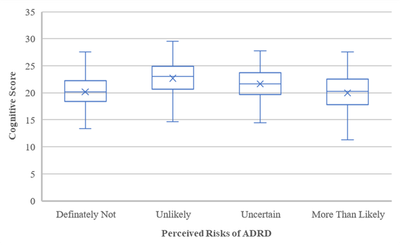## How to obtain outcome values among categorical exposures in a mixed model

Hi everyone,

I hope you are doing well. I am trying to get outcome values among categorical exposures in a mixed model.

Exposure in this study is the perceived risk of ADRD.

Outcome is the cognitive score.

I want to get the figure below but I do not know how to get outcome values in a mixed model.here is my code:

proc mixed covtest noclprint data = hrslong02 method=REML PLOTS(MAXPOINTS=none );
class hhidpn percived(ref="Unlikely") RAGENDER(ref="Female") race(ref="1") edu_4cat(ref="Lt High-school") married(ref="1") emply(ref="1");
model cogtot = percived visit_time percived*visit_time age10 RAGENDER race edu_4cat married emply hhincomelog_baseline  / solution cl ddfm = SATTERTHWAITE;
random intercept visit_time / subject=hhidpn type=un ;
run;

## Re: How to obtain outcome values among categorical exposures in a mixed model

By "outcome values", do you mean predicted values? if so, you would add the OUTP=preddata option in the MODEL statement in PROC MIXED. Then the preddata data set would contain the predicted values.

Hope this helps,

Jill

Discussion stats